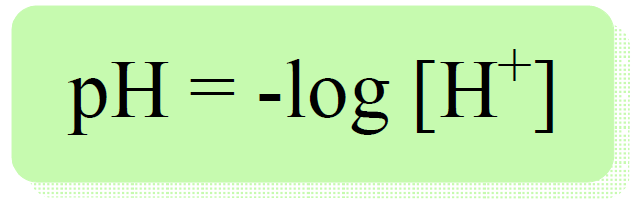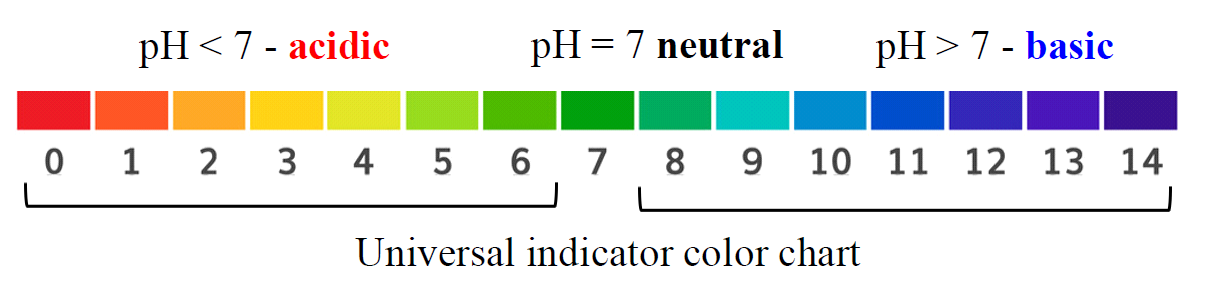## General Chemistry

Because the concentrations of H+ and OH ions in aqueous solutions are usually very small, to specify the acidity of a solution with more convenient numbers, a quantity called pH is used.

The pH is defined as the negative logarithm of proton/hydronium ion concentration:For example, if [H+] = 10-4 mol/l, the pH will be:

pH = -log 10-4 = 4 (acidic)

How do we know it is an acidic solution?

Remember, from the dissociation of water that Kw = [H+][OH] = 10-14 and if [H+] > 10-7 M, then the solution is acidic, and if [H+] < 10-7 M, we have a basic solution.

So, let’s say we have a basic solution with [OH] = 10-5. The [H+] can be calculated from Kw:

${\rm{[}}{{\rm{H}}^{\rm{ + }}}{\rm{]}}\;\;{\rm{ = }}\;\frac{{\sqrt {{K_{\rm{w}}}} \;}}{{{\rm{[O}}{{\rm{H}}^{\rm{ – }}}{\rm{]}}}}\; = \;\frac{{{{10}^{ – 14}}\;}}{{{{10}^{ – 5}}\;}}\, = 1.0\, \times \,{10^{ – 9}}\,M$

And the pH, therefore, is:

pH = -log 10-9 = 9 (basic)

Now, for a neutral solution, the pH is 7 because a solution is neutral when [H+] = [OH] = 10-7 M, and the pH in this case is:

pH = -log 10-7 = 7 (neutral)

So, the pattern is; if pH < 7, the solution is acidic, if pH = 7, the solution is neutral, and if pH > 7, we have a basic solution.

The pH scale goes from 0 to 14. The smaller the pH, the more acidic the solution.# The pOH and Basicity

Although, not as commonly used, the pOH values also describe the acidity/basicity of the solution. It is again the negative log which is a convenient method for expressing small numbers.

pOH = – log [OH]

Example:

Calculate the pOH of a solution with [OH] = 10-2 (basic).

pOH = – log [OH] = -log 10-2 = 2

Like in the pH scale, a solution is neutral if pOH = 7.  A pOH less than 7 is basic and a pOH greater than 7 is acidic.

In the next few post, we will discuss the relationship between pH and pOH and how it is used to calculate the pH and pOH from one another.

Check Also